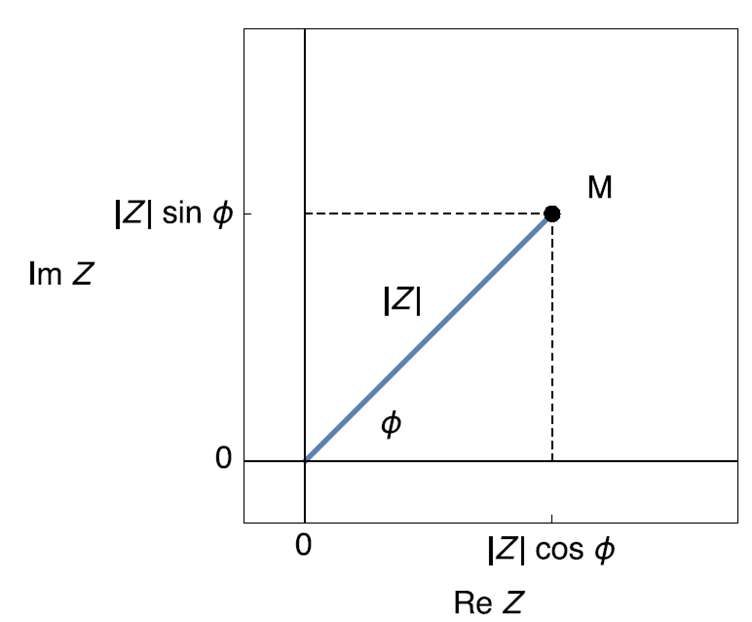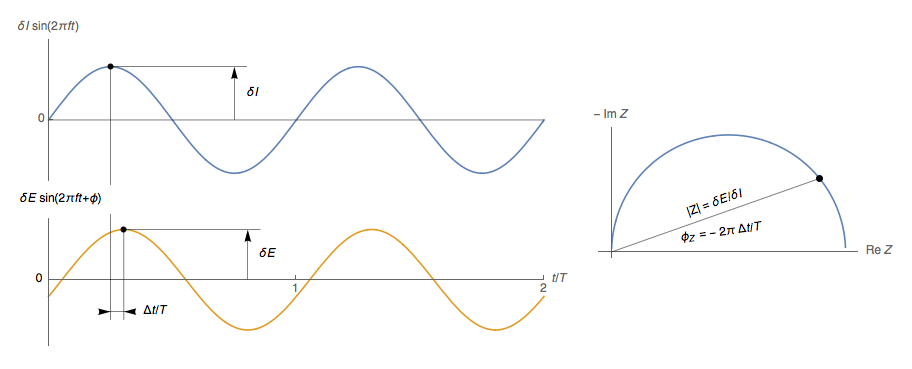Topic 10 min read# What is the definition of the impedance Z?

Latest updated: February 2, 2022

## The definition of impedance Z

Electrochemical Impedance Spectroscopy (EIS) is a powerful tool enabling the study of processes that occur at the interface of an electrode. It is extremely useful and is relevant to a very wide range of applications . But it can be difficult to define, and sometimes erroneous definitions are given. In this article we will try to give simple definitions of $Z$, which is the symbol commonly used for impedance.

It is well known that the response of a linear time invariant (LTI) system to a sine wave stimulation $\delta I \sin(2 \pi f t)$ with amplitude $\delta I$ and frequency $f$ is also a sine wave of the $f$ frequency with phase-shift of $\phi$ with $\phi= 2 \pi \Delta t/T$ as shown in Fig. 1 .Figure 1: Potential response of an LTI system to a current stimulation,  $T=1/f$, $\phi= 2 \pi \Delta t/T$.

## An incorrect definition of impedance

It is sometimes claimed in the literature that the impedance $Z$ of an electrochemical system can be calculated for each frequency in a method analogous to Ohm’s law:

$$Z=\frac{\delta E \sin(2\pi f t+ \phi)}{\delta I \,\sin(2\pi f t )}\;\;\;\; (1)$$

Since physical quantities $\delta E$, $\sin(2\pi f t)$, $\delta I$, and $\sin(2\pi f t + \phi)$ are real numbers, the impedance defined according to Eq. (1)  is also a real number. This definition (1) is too simple and therefore erroneous since an impedance is a complex quantity [3,4]. There are several better ways to introduce and define impedance.

## Definition using polar coordinates

Using Fig. 1 it is possible to define the modulus of $Z$: $|Z| = \delta E/\delta I$ and its phase $\phi= 2 \pi \Delta t/T$. A modulus and a phase define the polar coordinates of a point M in the plane (Fig. 2) . The polar coordinates $|Z|$ and $\phi$ can be converted into Cartesian coordinates $\text{Re}\;Z$ and $\text{Im}\;Z$ using the trigonometric functions sine and cosine:

$$\text{Re}\;Z = |Z| \cos \phi,\; \text{Im}\;Z = |Z| \sin\phi,\;\phi=\arctan\left(\frac{\text{Im}\;Z}{\text{Re}\;Z}\right) \;\;\;\; (2)$$

which can be written in complex notation

$$Z =\text{Re}\;Z+j \;\text{Im}\;Z= |Z| (\cos \phi+ j \sin \phi) \;\;\;\; (3)$$

where $j=\sqrt{-1}$.Figure 2 : Image of a complex number in the complex plane.

## Definition using an analytical signal

Another method of defining impedance is to use a mathematical trick consisting of replacing real signals with complex analytical signals. Real signals $I(t)=\delta I \sin(2 \pi f t)$ and $E(t)=\delta E \sin(2 \pi f t+\phi)$ are replaced by complex signals $I(t)=\delta I (\cos(2 \pi f t)+j\sin(2 \pi f t))$ and $E(t)=\delta E (\cos(2 \pi f t+\phi)+j\sin(2 \pi f t+\phi))$ which allow the impedance to be written correctly according to (4):

$$Z=\frac{\delta E (\cos(2 \pi f t+\phi)+j\sin(2 \pi f t+\phi))}{ \delta I (\cos(2 \pi f t)+j\sin(2 \pi f t))}=\frac{\delta E \exp(j(2 \pi f t+\phi))}{\delta I \exp(j(2 \pi f t))}=\frac{\delta E}{\delta I}\exp(j\phi) \;\;\;\; (4)$$

Which can be compared to (1).

## Definition using Laplace transform

A final and more general way of defining impedance involves the Laplace transforms of the input and output signals .

Finally, the change of $|Z|$ and $\phi$ with $f$ is shown in Fig. 3 for a circuit R/C.Figure 3: Evolution of the impedance of an R/C circuit $Z=R/(1+ \tau j 2 π f)$ with the frequency $f$.

References

 Topic What is EIS ?

 Application Note 50 – 1 The simplicity of complex numbers

 Application Note 50 – 2 The simplicity of Laplace transform

Electrochemical Impedance Spectroscopy EIS Impedance analytical signal Laplace transform